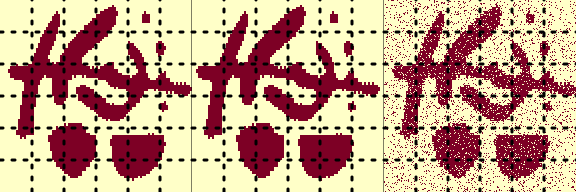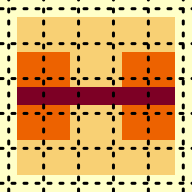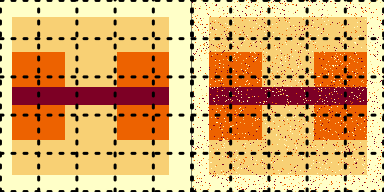# Two-Dimensional Examples of CatSIM

library(catsim)

## Introduction to CatSIM

The Categorial Structural Similarity Image Metric, or CatSIM, provides a similarity metric for binary or multinary images or maps after the style of MS-SSIM. That is, CatSIM compares two different sets of labels of pixels (voxels) in 2-D (or 3-D) images in an image. It attempts to assess similarity between two images in a way that accounts for the structural features of the image rather than a per-pixel discrepancy, ideally doing so similarly to human assessments of similarity. The package implements the methods described in (Thompson and Maitra 2020).

## How CatSIM works

CatSIM computes a set of similarity metrics on a sliding window in the images, then averages and combines them. In then downsamples the images and repeats the process. Finally, it combines the measures from the different levels together. It can use a variety of different similarity metrics inside of it depending on the specific meaning of the labels in the image. By default, it uses Cohen’s kappa inside it as its main comparison between the two images. However, there are several other options available which are more appropriate in some contexts.

## Constructing a binary illustration

Besag (1986) presents a $$100 \times 88$$ two-dimensional two-class image with “intentionally awkward” features. Here we blow it up to three times its size and create two distorted versions to demonstrate how the method works.

The first distorted version is shifting the body of the figure over 6 pixels. The second distorted version is adding salt-and-pepper noise so the error rate matches the difference between original and the shifted version.

set.seed(20200308)

multfactor <- 3
onesmat <- matrix(1, multfactor, multfactor)
exmat <- (besag %% 2) ### converting to binary - it's ones and twos

bigmat <- exmat %x% onesmat

bigmat <- exmat %x% onesmat
shift <- 6
basemat <- bigmat[1:(96 * multfactor), 1:(84 * multfactor)]
shiftmat <- bigmat[(1 + shift):(96 * multfactor + shift), 1:(84 * multfactor)]
acc <- mean(basemat == shiftmat)
errmat <- (matrix(
sample(0:1, 96 * 84 * (multfactor^2),
replace = TRUE, prob = c(acc, ((1 - acc)))
),
(96 * multfactor), (84 * multfactor)
) + basemat) %% 2
par(mfrow = c(1, 3), mar = c(0, 0, 0, 0))
image(basemat, xaxt = "n", yaxt = "n")
grid(lwd = 3, nx = 6, col = "black")
image(shiftmat, xaxt = "n", yaxt = "n")
grid(lwd = 3, nx = 6, col = "black")
image(errmat, xaxt = "n", yaxt = "n")
grid(lwd = 3, nx = 6, col = "black")Here we have the original, the shifted version, and the salt-and-pepper noise. We argue that though the proportion of pixels that differ from the base image are approximately the same for the two, an image similarity metric should say the shift is better.

catsim(basemat, shiftmat)
#>  0.5648881

catsim(basemat, errmat)
#>  0.4614073

As we might desire, the catsim metric judges the shift to be more like the original than the salt-and-pepper noise.

Because this is a two-class image, you can consider using the Jaccard index as the metric inside it with similar results:

catsim(basemat, shiftmat, method = "Jaccard")
#>  0.5647088

catsim(basemat, errmat, method = "Jaccard")
#>  0.52999

## Constructing a multiclass example

To demonstrate how it works, first we construct a simple multicategory image and blow it up to a larger size. The grid lines are added for reference. This is our “ground truth” image.


multfactor <- 20
onesmat <- matrix(1, multfactor, multfactor)
exmat <- matrix(0, 12, 12)
exmat[2:10, c(2, 3, 9, 10)] <- 1
exmat[2:10, c(4, 5, 7, 8)] <- 2
exmat[5:7, 2:10] <- 1
exmat[2:10, c(6)] <- 3
par(mar = c(0, 0, 0, 0))
image(exmat[11:1, 1:11], xaxt = "n", yaxt = "n")
grid(col = "black", lwd = 3)bigmat <- exmat %x% onesmat

Next, we construct two distorted versions. The first simply shifts the central portion of the image over six pixels. Then, we compute the percentage of pixels that disagree with the baseline image and create a second distorted image by adding salt-and-pepper noise at that rate. Again, grid lines are added only for reference.

set.seed(20200323)
shift <- 6 # we are shifting horizontally by six pixels
basemat <- bigmat[1:(11 * multfactor), 1:(11 * multfactor)]
shiftmat <- bigmat[(1 + shift):(11 * multfactor + shift), 1:(11 * multfactor)]
### Here we have shifted the matrix slightly
par(mfrow = c(1, 2), mar = c(0, 0, 0, 0))
image(shiftmat, xaxt = "n", yaxt = "n")
grid(col = "black", lwd = 3)

### computing the error rate
acc <- mean(basemat == shiftmat)
errmat <- (matrix(
sample(0:3, 121 * (multfactor^2),
replace = TRUE,
prob = c(acc, rep((1 - acc) / 3, 3))
),
(11 * multfactor), (11 * multfactor)
) + basemat) %% 4

### here we have made an image that matches its accuracy with salt and pepper noise
image(errmat, xaxt = "n", yaxt = "n")
grid(col = "black", lwd = 3)Depending on your preferences, you may have reason to prefer one or the other, but, arguably, the shifted version is in some sense better, even though their pixelwise agreement is approximately the same.

## Computing Image Similarity

If we take only one level of catsim, we get the following results when comparing to the initial matrix:

library(catsim)
### comparing base to shifted
catsim(basemat, shiftmat, weights = 1)
#>  0.6860161

### comparing base to salt-and-pepper
catsim(basemat, errmat, weights = 1)
#>  0.105825

### looking at accuracy
mean(basemat == shiftmat)
#>  0.9355372
mean(basemat == errmat)
#>  0.9364463

This captures the similarity between the original image and the shifted image, but is too “critical” of the salt-and-pepper error. We can correct this by looking at the image on multiple scales: we downsample by a factor of 2 and then compute the index for that layer, then repeat, and combine the layers at the end into one measure. By default, we use 5 layers of downsampling, but this can be specified based on your application and the size of the image. Downsampling should remove and smooth out a light level of salt-and-pepper error.

With five levels, the difference is smaller, but still prefers the shifted version over the salt and pepper error.

catsim(basemat, shiftmat, weights = rep(.2, 5))
#>  0.7525661

### comparing base to salt-and-pepper
catsim(basemat, errmat, weights = rep(.2, 5))
#>  0.5724128

## CatSIM options

By default, CatSIM uses Cohen’s kappa ($$\kappa$$) as the similarity comparison for the images. This measures agreement between the classes with and adjustment for the probability of chance agreement. However, depending on the application, a different comparison might make sense. The following options are available (using the method argument):

• Jaccard index, suitable for binary images where presence of one class is important (for instance, fMRI activation maps), with method="Jaccard".
• Dice index, which is similar to the Jaccard index, with method="Dice".
• Rand index, a similarity metric for comparing clusterings based on whether pairs of points are in the same group, with method="Rand".
• Adjusted Rand index, a modification of the Rand index which corrects for the probability of chance agreements, with method ="AdjRand" or ARI.
• Accuracy, or pixel-wise agreement, which is simply (# pixels agreeing)/(# pixels), with method="accuracy".
• Normalized mutual information, a information theory similarity metric based on the Kullback-Liebler divergence between the two distributions, with method = "NMI" or MI.
• Adjusted mutual information, a correction to the mutual information to account for the probability of chance agreements, with method = "AMI".
catsim(basemat, shiftmat, weights = rep(.5, 2), method = "Rand")
#>  0.7972214
catsim(basemat, shiftmat, weights = rep(.5, 2), method = "NMI")
#>  0.2701349

The window size can also be adjusted. It should be large enough to capture the scale of the features that are relevant in the analysis. In this example, a portion of the image was displaced by 6 pixels. Too large of a window may average out that difference too much while too small may miss the similarity between the images. Here is a comparison between the shifted image and the salt-and-pepper noise at different window sizes.

catsim(basemat, shiftmat, weights = rep(1 / 3, 3), window = 20)
#>  0.7878624
catsim(basemat, shiftmat, weights = rep(1 / 3, 3), window = 5)
#>  0.7424498
catsim(basemat, errmat, weights = rep(1 / 3, 3), window = 20)
#>  0.6766773
catsim(basemat, errmat, weights = rep(1 / 3, 3), window = 5)
#>  0.441829

The number of levels CatSIM uses can be set by specifying either the levels argument or passing a vector to weights, where the length of the vector dictates how many levels of downsampling will be performed. By default, if the number of levels are specified without specifying the weights, the weights are set to be equal across levels and add up to 1. Specifying weights = rep(1/3,3) is the same as specifying levels = 3.

The catsim() function works for both 2-D and 3-D images. It is a wrapper for other functions which give more control over the various options. On a 2-D image, it is calling catmssim_2d() and on a 3-D image it is calling either catmssim_3d_cube() or catmssim_3d_slice(), which treats the image as either an isotropic 3-D image or a stack of 2-D slices.
In images with different properties across dimensions, the sliding window used to assess the similarity might not need to be square. This can be specified by passing a vector to the window argument. In the provided examples, this is not necessary, but some sources of images may not be isotropic. Alternatively, the image may be elongated in one direction and narrow in another. This makes it possible to accommodate these differences.
catsim(basemat, shiftmat, weights = rep(.2, 5), window = c(11, 5))
#>  0.7299104